Miscellaneous

Chapter 6 Class 12 Application of Derivatives
Serial order wise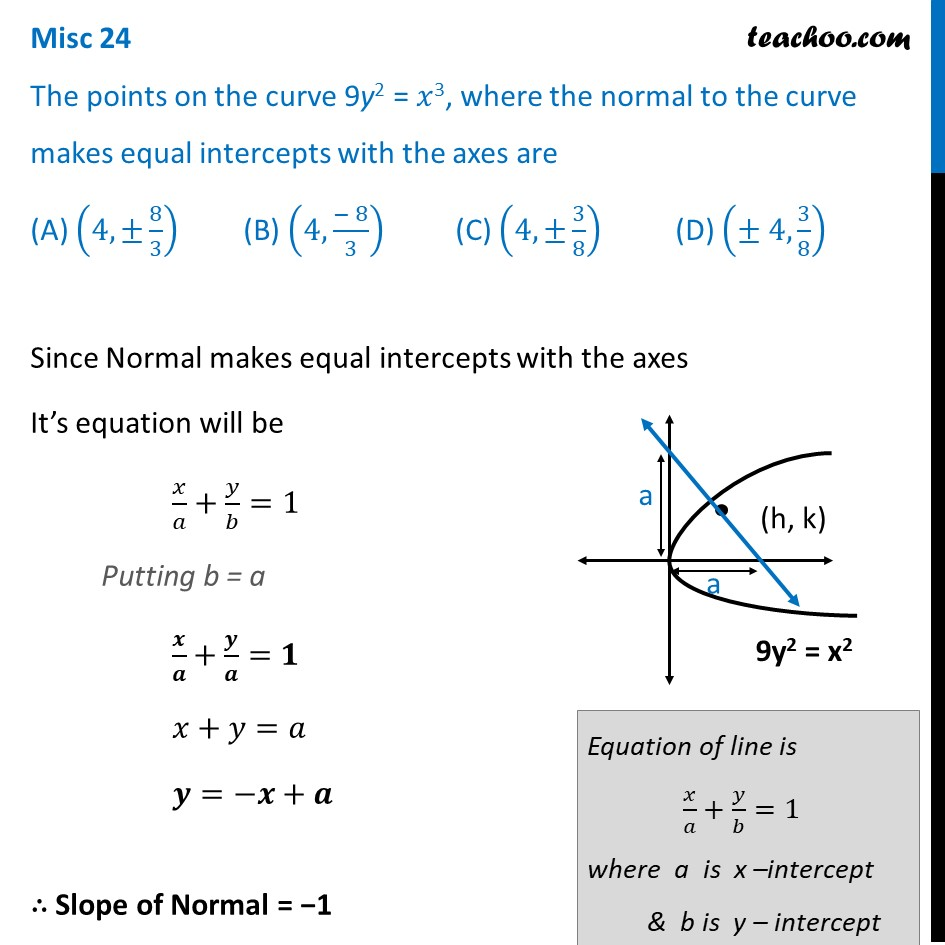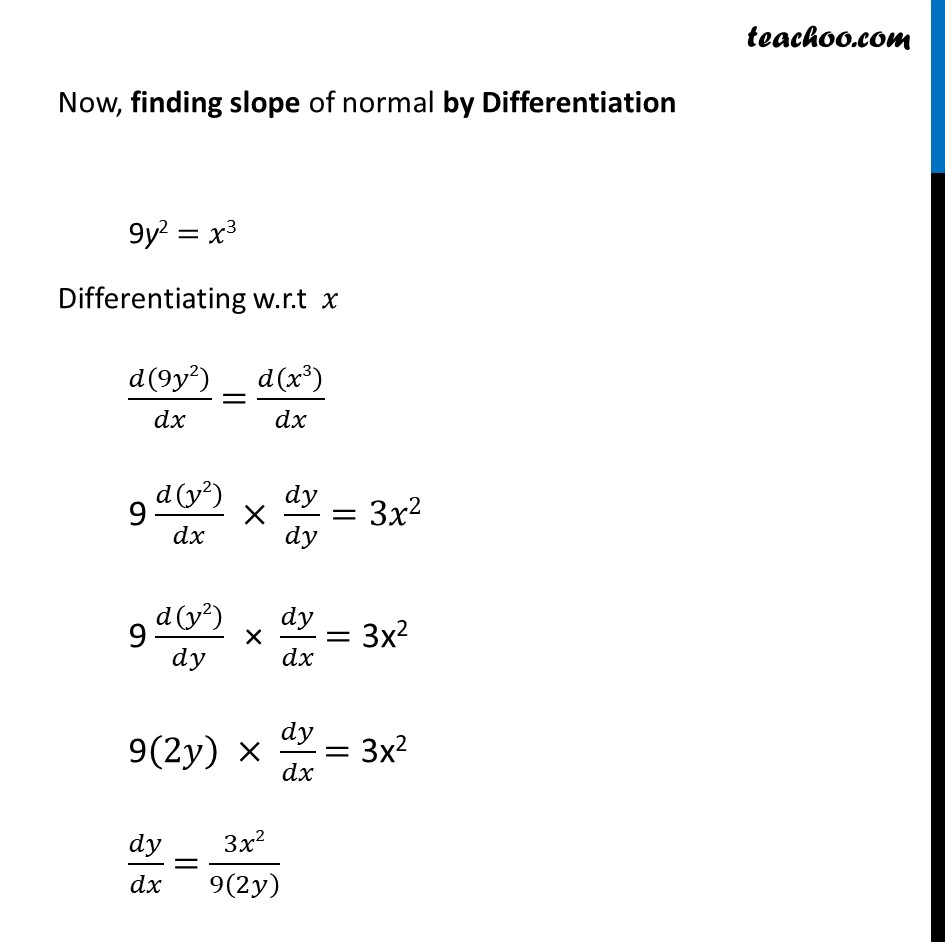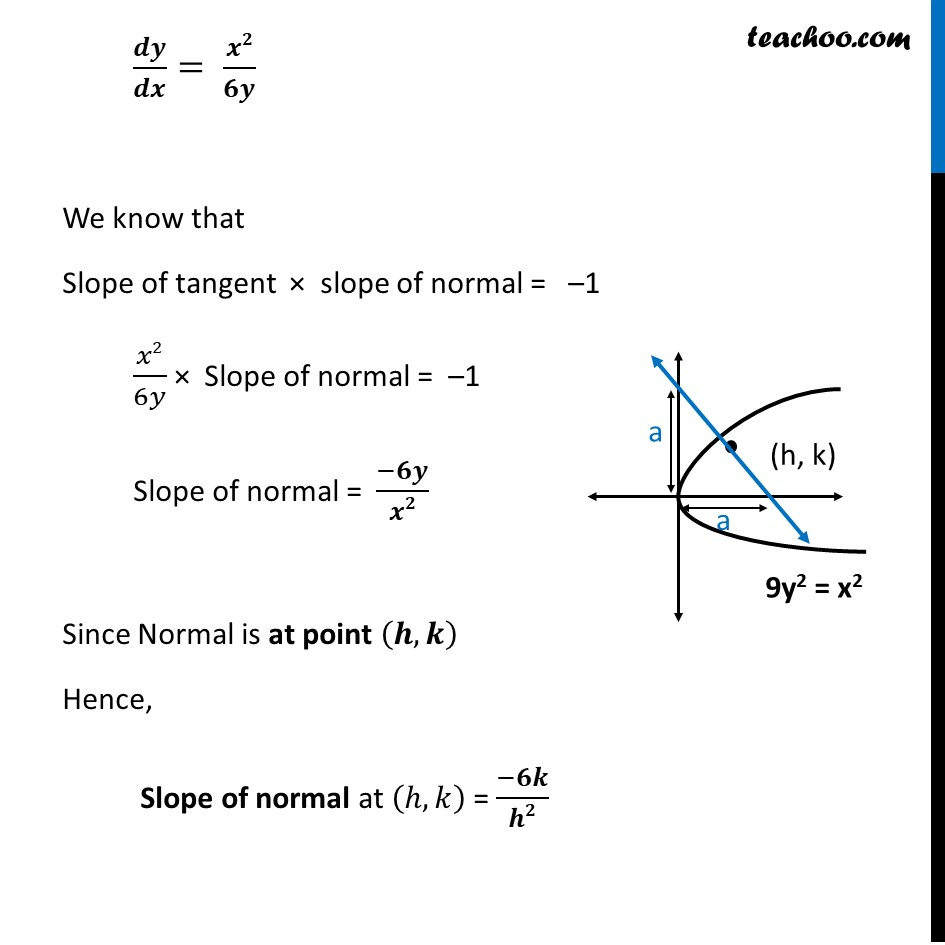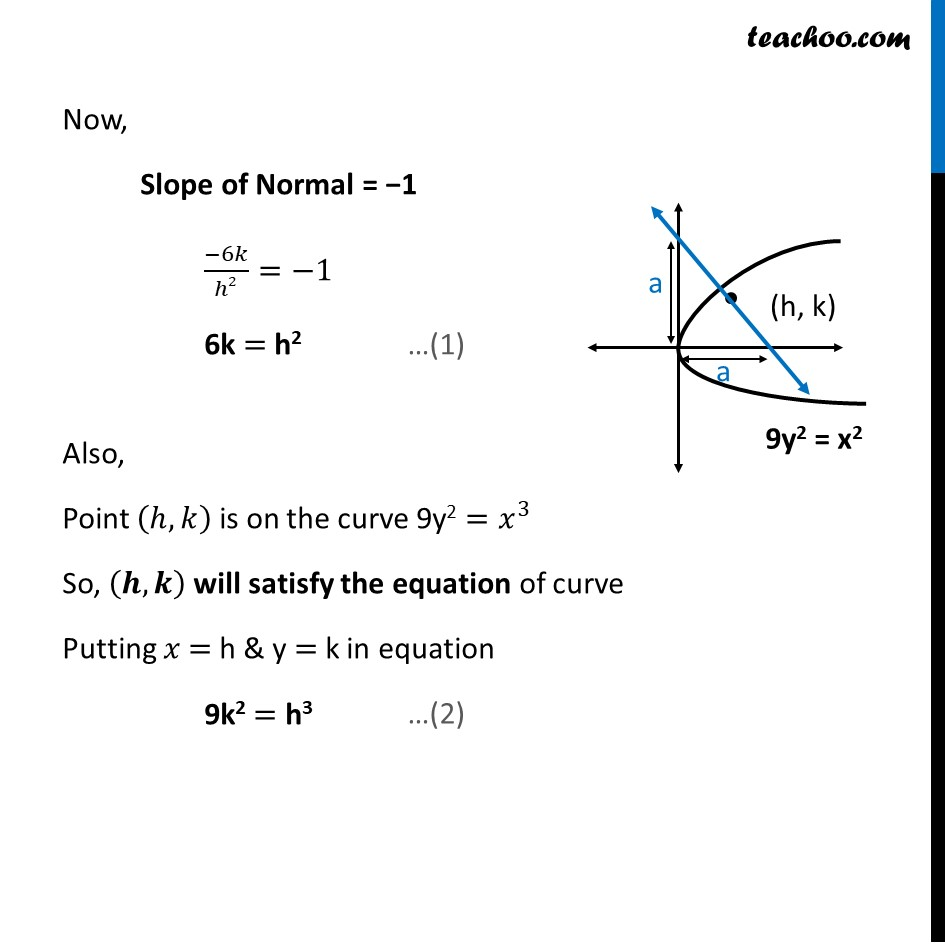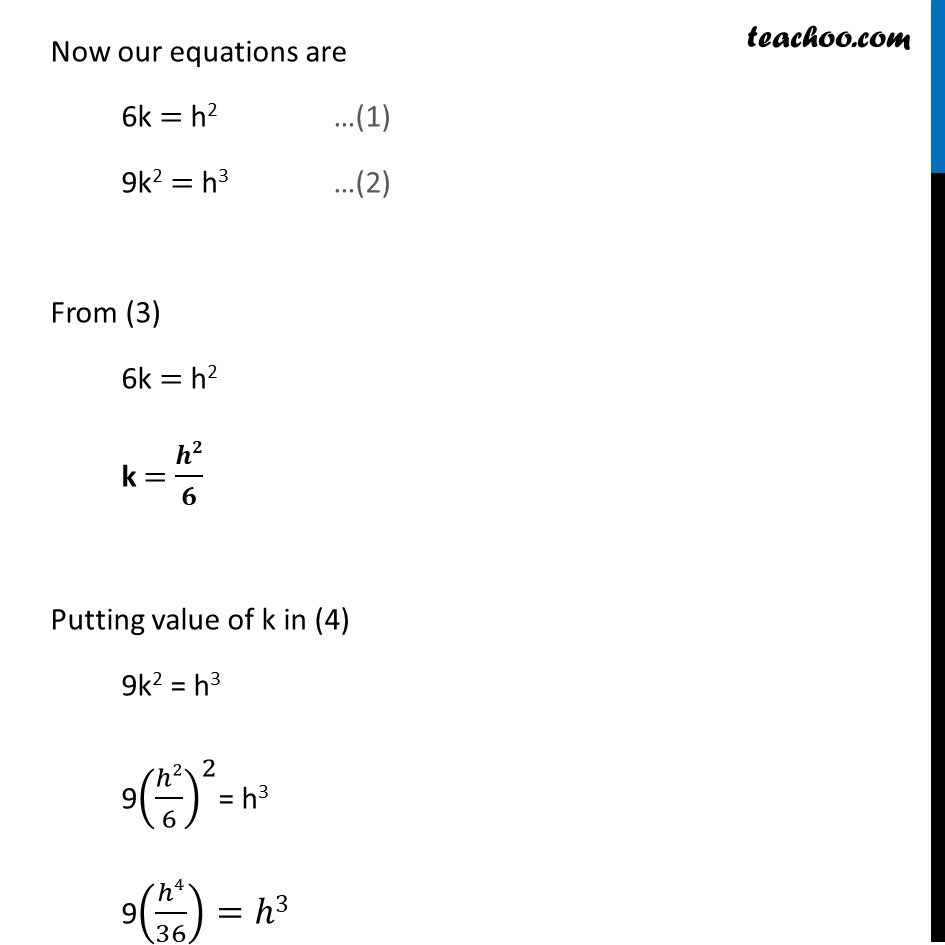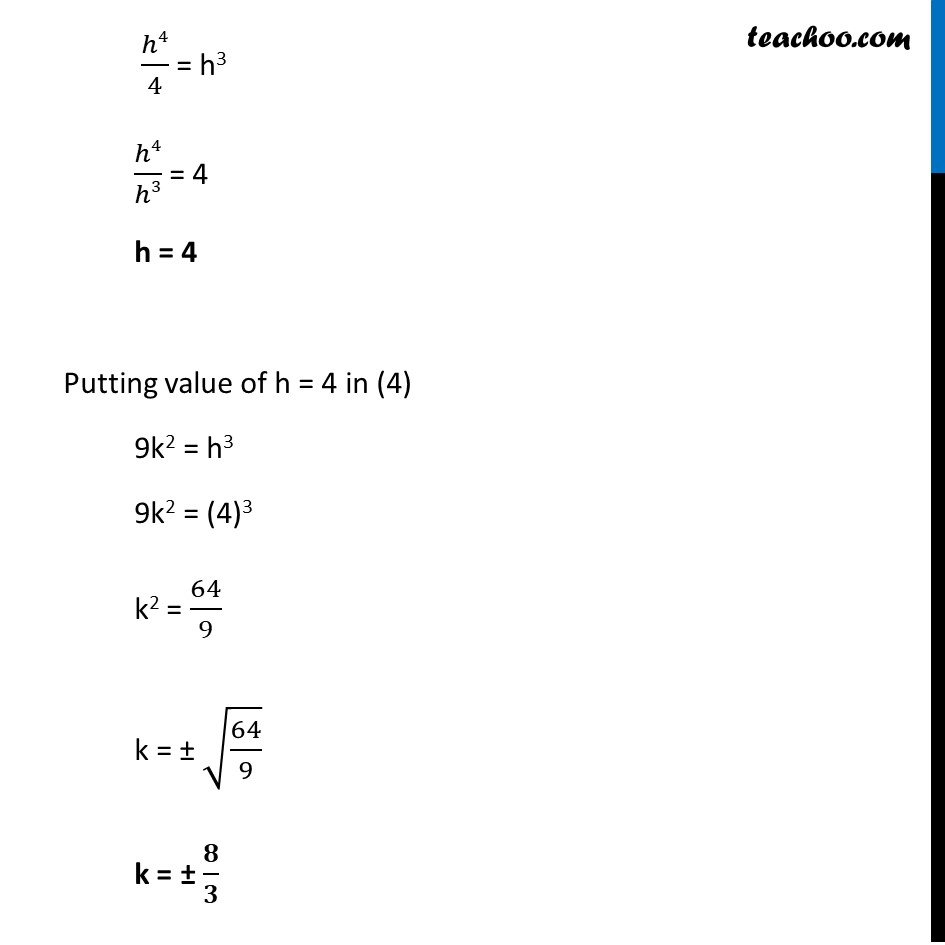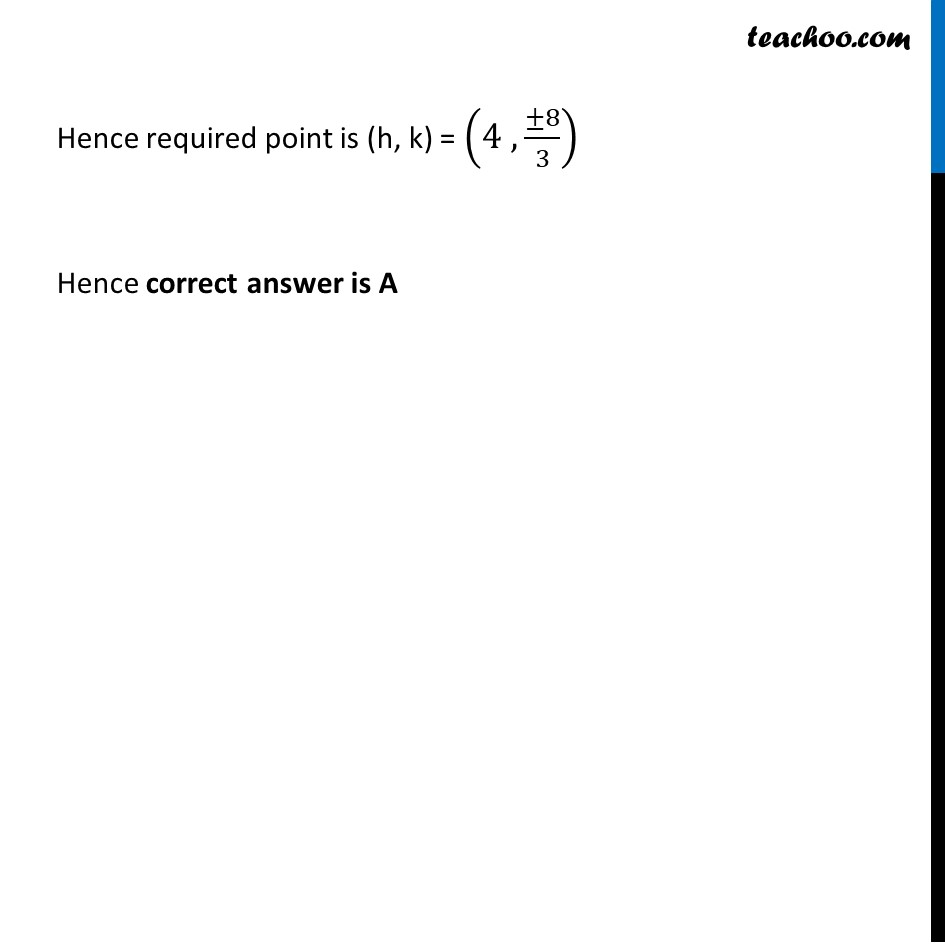Learn in your speed, with individual attention - Teachoo Maths 1-on-1 Class

### Transcript

Misc 24 The points on the curve 9y2 = 𝑥3, where the normal to the curve makes equal intercepts with the axes are (A) (4,±8/3) (B) (4,(− 8)/3) (C) (4,±3/8) (D) (± 4, 3/8) Since Normal makes equal intercepts with the axes It’s equation will be 𝑥/𝑎+𝑦/𝑏=1 Putting b = a 𝒙/𝒂+𝒚/𝒂=𝟏 𝑥+𝑦=𝑎 𝒚=−𝒙+𝒂 ∴ Slope of Normal = −1 Equation of line is 𝑥/𝑎+𝑦/𝑏=1 where a is x –intercept & b is y – intercept Now, finding slope of normal by Differentiation 9y2 = 𝑥3 Differentiating w.r.t 𝑥 𝑑(9𝑦2)/𝑑𝑥 = 𝑑(𝑥3)/𝑑𝑥 9 𝑑(𝑦2)/𝑑𝑥 × 𝑑𝑦/𝑑𝑦=3𝑥2 9 𝑑(𝑦2)/𝑑𝑦 × 𝑑𝑦/𝑑𝑥 = 3x2 9(2𝑦) × 𝑑𝑦/𝑑𝑥 = 3x2 𝑑𝑦/𝑑𝑥 = 3𝑥2/9(2𝑦) 𝒅𝒚/𝒅𝒙= 𝒙𝟐/𝟔𝒚 We know that Slope of tangent × slope of normal = –1 𝑥2/6𝑦 × Slope of normal = –1 Slope of normal = (−𝟔𝒚)/𝒙𝟐 Since Normal is at point (𝒉,𝒌) Hence, Slope of normal at (ℎ,𝑘) = (−𝟔𝒌)/𝒉𝟐 Now, Slope of Normal = −1 (−6𝑘)/ℎ2=−1 6k = h2 Also, Point (ℎ,𝑘) is on the curve 9y2 =𝑥^3 So, (𝒉,𝒌) will satisfy the equation of curve Putting 𝑥 = h & y = k in equation 9k2 = h3 Now our equations are 6k = h2 …(1) 9k2 = h3 …(2) From (3) 6k = h2 k = 𝒉𝟐/𝟔 Putting value of k in (4) 9k2 = h3 9(ℎ2/6)^2= h3 9(ℎ4/36)=ℎ3 ℎ4/4 = h3 ℎ4/ℎ3 = 4 h = 4 Putting value of h = 4 in (4) 9k2 = h3 9k2 = (4)3 k2 = 64/9 k = ± √(64/9) k = ± 𝟖/𝟑 Hence required point is (h, k) = (4 , (±8)/3) Hence correct answer is A